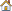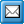﻿ GetServerModels
QPR Knowledge Base 2017.1

GetServerModelsDeveloper's Guide > QPR API > The Object Model > QPR Modeling > PGApplication Commands:

GetServerModelsGetServerModelsRevision history:

Introduced in QPR 7.2.1

Fetches a list of the server's models into a variant.

Synopsis:

PGApplication.GetServerModels(out Models)

Parameters:

out Models: Variant array for holding the list of model contained in the server. The array has one row for each model with the following column contents:

0: Name of a model, folder, or a template. String.

1: ID of the model, folder, or template. Integer.

2: ID of the folder where the model, folder, or template is located in. Integer.

3: Type (1 = folder, 2 = regular model, 3 = base model, 4 = template, 5 = model version root folder, 6 = model version branch folder, 7 = old revision of a model version, 8 = old revision of a base model version). Integer.

4: Published (0 = no, 1 = yes, only for models). Integer.

5: Modified date/time (only for models). Date(double)

6: Version (only for models). String.

7: Author (only for models). String.

8: Comment (only for models). String.

9: Full path of the model. String.

10: Feedback address (only for models). String.

11: Status (only for models). Integer.

12: Internal version number (only for models). Integer.

13: Branch. String. If the model is inside a branch, the branch name is returned here.

Required Rights:

None. This function returns all the models the user has rights to.

Return Values:

Below are listed the return values that this function can return:

RV_SUCCESS

RV_APPLICATION_QUIT_CALLED

RV_NOT_CONNECTED_TO_SERVER

RV_NOT_AUTHENTICATED

Example Procedure:

Sub GetServerModels()

Dim iResult

Dim avModels

Dim sMessage

Dim i

Call ConnectToServerAndAuthenticate

iResult = PGApplication.GetServerModels(avModels)

If (iResult <> 0) Then

MsgBox "Get server models failed: " + PGApplication.GetErrorMessage(iResult)

End If

sMessage = "Models in server:" + vbLf + vbLf

For i = 0 To UBound(avModels, 1)

If (avModels(i, 3) = 1) then

sMessage = sMessage + "Folder: " + avModels(i, 0) + vbLf

End If

If (avModels(i, 3) = 2) then

sMessage = sMessage + "Model: " + avModels(i, 0) + vbLf

End If

If (avModels(i, 3) = 3) then

sMessage = sMessage + "Base model: " + avModels(i, 0) + vbLf

End If

If (avModels(i, 3) = 4) then

sMessage = sMessage + "Template: " + avModels(i, 0) + vbLf

End If

Next

MsgBox sMessage

End Sub